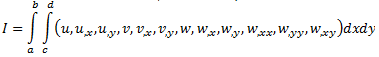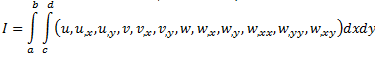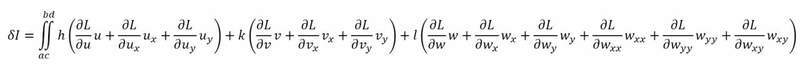# Calculation boundary terms of a functional

ronaldinho52
Dear all,

I am stuck with the problem which is given below;In this problem the equilibrium equations of the given functional must be derived in u, v, and w directions from which the boundary terms must be found. I think that i have derived the equilibrium equations( 5 equations), but i dont know how to proceed. Does anyone maybe know how to do it??

#### Attachments

Homework Helper
Dear all,

I am stuck with the problem which is given below;In this problem the equilibrium equations of the given functional must be derived in u, v, and w directions from which the boundary terms must be found. I think that i have derived the equilibrium equations( 5 equations), but i dont know how to proceed. Does anyone maybe know how to do it??

I think you're missing a name for the integrand. I will use $L$.

To determine $\delta I$, integrate $L(u + h, \dots, v + k, \dots, w + l, \dots) - L(u, \dots, v, \dots, w, \dots)$ term by term. You can swap the order of integration so that terms involving derivatives with respect to x are integrated with respect to x first, and terms involving derivatives with respect to y are integrated with respect to y first.

For example, for the $u_{,x}$ term you get:
$$\int_a^b \int_c^d h_{,x} \frac{\partial L}{\partial u_{,x}}\,\mathrm{d}x \,\mathrm{d}y = \int_a^b \left[ h \frac{\partial L}{\partial u_{,x}}\right]_c^d\,\mathrm{d}y - \int_a^b \int_c^d h \frac{\partial}{\partial x} \left(\frac{\partial L}{\partial u_{,x}}\right)\,\mathrm{d}x\,\mathrm{d}y$$

(The antiderivative with respect to x of $h_{,x}$ is $h + A(y)$ for an arbitrary function $A$; but it will be the same function at both $x = c$ and $x = d$, so it cancels out.)

Ideally the conditions of your problem are such that the perturbation $h$ vanishes everywhere on the boundary, so the boundary term vanishes.

With the second derivatives of w you have to integrate by parts twice:
$$\int_a^b \int_c^d l_{,xx} \frac{\partial L}{\partial w_{,xx}}\,\mathrm{d}x \,\mathrm{d}y = \int_a^b \left[ l_{,x} \frac{\partial L}{\partial w_{,xx}} \right]_c^d\,\mathrm{d}y - \int_a^b \int_c^d l_{,x} \frac{\partial}{\partial x}\left(\frac{\partial L}{\partial w_{,xx}}\right)\,\mathrm{d}x \,\mathrm{d}y$$
Again, one would hope that the conditions of your problem require that $l_{,x}$ vanishes on the boundary, so the boundary term vanishes. The remaining term is dealt with as for the other first derivatives.

Incidentally, I believe there should be three equilibrium equations (one each for u, v and w) so if you have five then you have made an error somewhere.

ronaldinho52
I will try to solve the question now with your explanation. Thank you very much!!

ronaldinho52
Regarding your explanation I ended up with the following equation to be solved.Is this correct or did I get your explanation totally wrong??(btw i forgot the dxdy term at the end of the equation)